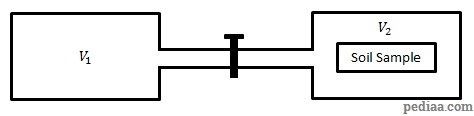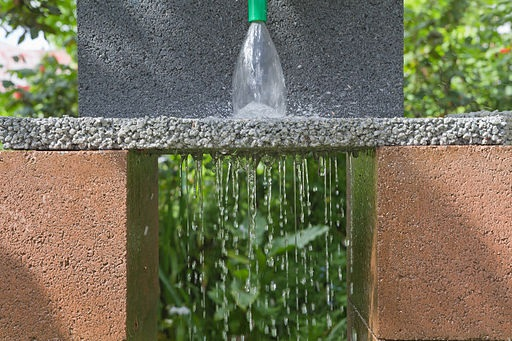# Difference Between Porosity and Permeability

## Main Difference – Porosity vs. Permeability

Porosity and permeability are both properties of rocks and soil. The main difference between porosity and permeability is that porosity is a measurement of space between rocks whereas permeability is a measurement of how easy it is for fluids to flow between rocks.

## What is Porosity

Porosity measures how much space is between rocks. It is taken to be the ratio of the volume of empty space (or pores) in a rock to its total volume, and it is often expressed in terms of a fraction or a percentage. Since fluids can occupy these free spaces between rocks, porosity is also a measurement of how much fluids that rocks can hold.

There are several methods to measure the porosity of a sample of soil. One method used to measure porosity is called the Archimedes method. To test porosity using this method, the mass of a sample of soil is first measured when the soil is dry. Let us call this mass$m_{\mathrm{dry}}$. Then, water is added to the sample until the soil sample is saturated and the mass of the soil sample and water,$m_{\mathrm{wet}}$ is measured. Next, the saturated sample is immersed in water, and the “mass” of the submerged sample,$m_{\mathrm{submerged}}$ is measured again. (Note that in fact we are measuring weights, however, the factors of$g$ cancel out, so you can treat the mass readings taken from a spring balance as readings of weight).

Then, the mass of water added to the sample is$m_{\mathrm{wet}}-m_{\mathrm{dry}}$. If the density of water is$\rho_w$, then the volume of pores in the soil sample is$v_{\mathrm{pores}}=\rho_w\left( m_{\mathrm{wet}}-m_{\mathrm{dry}}\right)$.

The total volume of soil is the volume of the fluid displaced when the sample is submerged in water. From the Archimedes’ Principle, the total volume is given by$v_{\mathrm{total}}=\rho_w\left( m_{\mathrm{wet}}-m_{\mathrm{submerged}}\right)$. Then, the porosity$\phi$ is given by$\phi=\frac{v_{\mathrm{pores}}}{v_{\mathrm{total}}}$. Therefore,$\phi=\frac{m_{\mathrm{wet}}-m_{\mathrm{dry}}}{m_{\mathrm{wet}}-m_{\mathrm{submerged}}}$

Another method for porosity measurement involves the use of Boyle’s Law. In this method, gases are used to fill up the pores between soil particles. According to Boyle’s law, for a fixed mass of gas at constant temperature the pressure of the gas is inversely proportional to its volume. To determine porosity using Boyle’s Law, two connected chambers are used. The volumes of these chambers are known and the pressure of gases inside these chambers can be measured using a pressure gauge.

Initially, the two chambers are separated by closing a valve between them. A container with a known volume, called the core, is filled with the soil sample. Let us call the volume of the core$V_{\mathrm{core}}$. The core is placed inside one of the chambers and  the air is taken out of this chamber. Gas is still present in the other chamber, and the air pressure inside this chamber is measured. Let us refer to the volume and pressure in this chamber as$V_1$ and$p_1$.Measuring Porosity using the Boyle’s Law method.

Next, the valve between the two chambers is opened, allowing for air from the first chamber to fill up the second chamber as well. The pressure in the chambers is measured again, and let this pressure be$p_2$. Using Boyle’s law, the total volume occupied by the gas, which we will call$V_f$, can now be calculated:$V_f=\frac{p_1V_1}{p_2}$

The volume$V_f$ can be given by,$V_f=V_1+V_2-V_{\mathrm{core}}+V_{\mathrm{pores}}$

where$V_2$ includes the volume of the second chamber and the tubing between the two chambers. Now, the above expression allows us to calculate the volume of pores$V_{\mathrm{pores}}$.$V_{\mathrm{core}}$ gives the total volume of the soil sample. The porosity$\phi$ is then given by,$\phi=\frac{V_{\mathrm{pores}}}{V_{\mathrm{core}}}$

## What is Permeability

Permeability is a measurement of how easily fluid can flow between rocks. A soil sample with a high permeability allows for liquids to flow through easily, whereas it is harder for liquids to flow through soil with low permeability. For instance, pavements can be constructed with a material with a high permeability so that water does not accumulate in puddles on the surface.Material forming “permeable pavements”.

Mathematically, permeability is described in Darcy’s law. Darcy’s law is a formula describing the flow rate of fluid through a porous medium. According to this law, if a fluid with a viscosity of$\mu$ flows through a volume of porous material with a cross-sectional area$A$ for a distance$L$, then the total volume of fluid discharged per second$Q$ is given by:$Q=-\frac{\kappa A \left( p_2-p_1\right)}{\mu L}$

where$p_1$ is the pressure at the start and$p_2$ is the pressure of fluid after flowing through length$L$.  The constant$\kappa$ in this formula is defined as the permeability of the medium. The unit of$\kappa$ is square metres (m2).

## Difference Between Porosity and Permeability

### What it Measures

Porosity measures the amount of empty space between rocks or in the soil as a fraction of the total volume.

Permeability measures how easy it is for fluids to flow through a substance.

### Units

Porosity is a ratio of volumes, so it has no units.

Permeability has units of area (m2 in the SI system).

Image Courtesy:

“A permeable paver demonstration, Austin’s Ferry, Tasmania, Australia” by JJ Harrison ([email protected]) (Own work) [CC BY-SA 3.0], via Wikimedia Commons